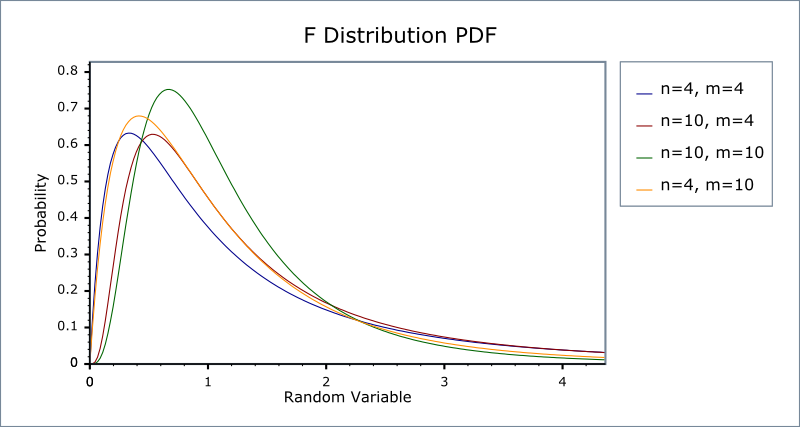#Boost C++ Libraries

...one of the most highly regarded and expertly designed C++ library projects in the world.

#### F Distribution

`#include <boost/math/distributions/fisher_f.hpp>`
```namespace boost{ namespace math{

template <class RealType = double,
class Policy   = policies::policy<> >
class fisher_f_distribution;

typedef fisher_f_distribution<> fisher_f;

template <class RealType, class Policy>
class fisher_f_distribution
{
public:
typedef RealType value_type;

// Construct:
fisher_f_distribution(const RealType& i, const RealType& j);

// Accessors:
RealType degrees_of_freedom1()const;
RealType degrees_of_freedom2()const;
};

}} //namespaces
```

The F distribution is a continuous distribution that arises when testing whether two samples have the same variance. If χ2m   and χ2n   are independent variates each distributed as Chi-Squared with m and n degrees of freedom, then the test statistic:

Fn,m   = (χ2n   / n) / (χ2m   / m)

Is distributed over the range [0, ∞] with an F distribution, and has the PDF:The following graph illustrates how the PDF varies depending on the two degrees of freedom parameters.##### Member Functions
```fisher_f_distribution(const RealType& df1, const RealType& df2);
```

Constructs an F-distribution with numerator degrees of freedom df1 and denominator degrees of freedom df2.

Requires that df1 and df2 are both greater than zero, otherwise domain_error is called.

```RealType degrees_of_freedom1()const;
```

Returns the numerator degrees of freedom parameter of the distribution.

```RealType degrees_of_freedom2()const;
```

Returns the denominator degrees of freedom parameter of the distribution.

##### Non-member Accessors

All the usual non-member accessor functions that are generic to all distributions are supported: Cumulative Distribution Function, Probability Density Function, Quantile, Hazard Function, Cumulative Hazard Function, mean, median, mode, variance, standard deviation, skewness, kurtosis, kurtosis_excess, range and support.

The domain of the random variable is [0, +∞].

##### Examples

Various worked examples are available illustrating the use of the F Distribution.

##### Accuracy

The normal distribution is implemented in terms of the incomplete beta function and its inverses, refer to those functions for accuracy data.

##### Implementation

In the following table v1 and v2 are the first and second degrees of freedom parameters of the distribution, x is the random variate, p is the probability, and q = 1-p.

Function

Implementation Notes

pdf

The usual form of the PDF is given by:However, that form is hard to evaluate directly without incurring problems with either accuracy or numeric overflow.

Direct differentiation of the CDF expressed in terms of the incomplete beta function

led to the following two formulas:

fv1,v2(x) = y * ibeta_derivative(v2 / 2, v1 / 2, v2 / (v2 + v1 * x))

with y = (v2 * v1) / ((v2 + v1 * x) * (v2 + v1 * x))

and

fv1,v2(x) = y * ibeta_derivative(v1 / 2, v2 / 2, v1 * x / (v2 + v1 * x))

with y = (z * v1 - x * v1 * v1) / z2

and z = v2 + v1 * x

The first of these is used for v1 * x > v2, otherwise the second is used.

The aim is to keep the x argument to ibeta_derivative away from 1 to avoid rounding error.

cdf

Using the relations:

p = ibeta(v1 / 2, v2 / 2, v1 * x / (v2 + v1 * x))

and

p = ibetac(v2 / 2, v1 / 2, v2 / (v2 + v1 * x))

The first is used for v1 * x > v2, otherwise the second is used.

The aim is to keep the x argument to ibeta well away from 1 to avoid rounding error.

cdf complement

Using the relations:

p = ibetac(v1 / 2, v2 / 2, v1 * x / (v2 + v1 * x))

and

p = ibeta(v2 / 2, v1 / 2, v2 / (v2 + v1 * x))

The first is used for v1 * x < v2, otherwise the second is used.

The aim is to keep the x argument to ibeta well away from 1 to avoid rounding error.

quantile

Using the relation:

x = v2 * a / (v1 * b)

where:

a = ibeta_inv(v1 / 2, v2 / 2, p)

and

b = 1 - a

Quantities a and b are both computed by ibeta_inv without the subtraction implied above.

quantile

from the complement

Using the relation:

x = v2 * a / (v1 * b)

where

a = ibetac_inv(v1 / 2, v2 / 2, p)

and

b = 1 - a

Quantities a and b are both computed by ibetac_inv without the subtraction implied above.

mean

v2 / (v2 - 2)

variance

2 * v22 * (v1 + v2 - 2) / (v1 * (v2 - 2) * (v2 - 2) * (v2 - 4))

mode

v2 * (v1 - 2) / (v1 * (v2 + 2))

skewness

2 * (v2 + 2 * v1 - 2) * sqrt((2 * v2 - 8) / (v1 * (v2 + v1 - 2))) / (v2 - 6)

kurtosis and kurtosis excess

 Copyright © 2006-2010, 2012, 2013 Paul A. Bristow, Christopher Kormanyos, Hubert Holin, Bruno Lalande, John Maddock, Johan Råde, Gautam Sewani, Benjamin Sobotta, Thijs van den Berg, Daryle Walker and Xiaogang Zhang Distributed under the Boost Software License, Version 1.0. (See accompanying file LICENSE_1_0.txt or copy at http://www.boost.org/LICENSE_1_0.txt)